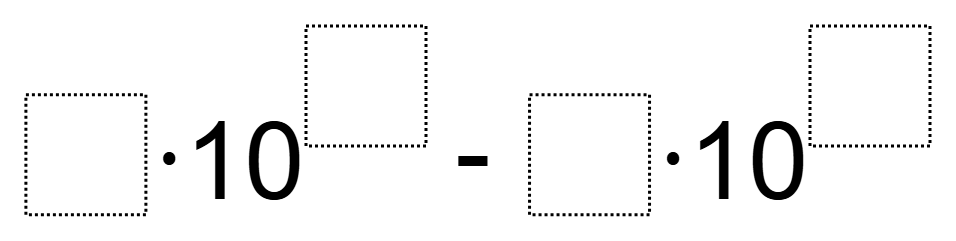Home > Grade 8 > Expressions & Equations > Differences in Scientific Notation

# Differences in Scientific Notation

Directions: Using the digits 1 to 9 at most one time each, fill in the boxes to make the largest (or smallest) absolute difference.### Hint

What are the biggest and smallest numbers you can make with these digits in scientific notation?
Try thinking of the numbers you create on a number line.

### Answer

Largest: 8×10^9 – 2×10^1
Smallest 3×10^2 – 9×10^1

Source: Marie Isaac

## Square Root Expression

Directions: Use the digits 1 to 9, at most one time each, to fill in …

### One comment

1.For smallest difference, one of my classes found the following:

1 x 10^3 – 9 x 10^2

1 x 1000 – 9 x 100

1000 – 900 = 100